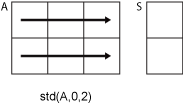# std

## 语法

``S = std(A)``
``S = std(A,w)``
``S = std(A,w,'all')``
``S = std(A,w,dim)``
``S = std(A,w,vecdim)``
``S = std(___,nanflag)``

## 说明

````S = std(A)` 返回 `A` 沿大小不等于 1 的第一个数组维度的元素的标准差。 如果 `A` 是观测值的向量，则标准差为标量。 如果 `A` 是一个列为随机变量且行为观测值的矩阵，则 `S` 是一个包含与每列对应的标准差的行向量。如果 `A` 是多维数组，则 `std(A)` 会沿大小不等于 1 的第一个数组维度计算，并将这些元素视为向量。此维度的大小将变为 `1`，而所有其他维度的大小保持不变。默认情况下，标准差按 `N-1` 实现归一化，其中 `N` 是观测值数量。 ```

``S = std(A,w)` 为上述任意语法指定一个权重方案。当 `w = 0` 时（默认值），`S` 按 `N-1` 进行归一化。当 `w = 1` 时，`S` 按观测值数量 `N` 进行归一化。`w` 也可以是包含非负元素的权重向量。在这种情况下，`w` 的长度必须等于 `std` 将作用于的维度的长度。 `
`当 `w` 为 0 或 1 时，`S = std(A,w,'all')` 计算 `A` 的所有元素的标准差。此语法适用于 MATLAB® R2018b 及更高版本。`

``S = std(A,w,dim)` 使用上述任意语法沿维度 `dim` 返回标准差。要维持默认归一化并指定操作的维度，请在第二个参数中设置 `w = 0`。`

`当 `w` 为 0 或 1 时，`S = std(A,w,vecdim)` 计算向量 `vecdim` 中指定维度的标准差。例如，如果 `A` 是矩阵，则 `std(A,0,[1 2])` 计算 `A` 中所有元素的标准差，因为矩阵的每个元素包含在由维度 1 和 2 定义的数组切片中。`

``S = std(___,nanflag)` 指定在上述任意语法的计算中包括还是忽略 `NaN` 值。例如，`std(A,'includenan')` 包括 `A` 中的所有 `NaN` 值，而 `std(A,'omitnan')` 则会忽略这些值。`

## 示例

```A = [4 -5 1; 2 3 5; -9 1 7]; S = std(A)```
```S = 1×3 7.0000 4.1633 3.0551 ```

```A(:,:,1) = [2 4; -2 1]; A(:,:,2) = [9 13; -5 7]; A(:,:,3) = [4 4; 8 -3]; S = std(A)```
```S = S(:,:,1) = 2.8284 2.1213 S(:,:,2) = 9.8995 4.2426 S(:,:,3) = 2.8284 4.9497 ```

```A = [1 5; 3 7; -9 2]; w = [1 1 0.5]; S = std(A,w)```
```S = 1×2 4.4900 1.8330 ```

```A = [6 4 23 -3; 9 -10 4 11; 2 8 -5 1]; S = std(A,0,2)```
```S = 3×1 11.0303 9.4692 5.3229 ```

```A(:,:,1) = [2 4; -2 1]; A(:,:,2) = [9 13; -5 7]; A(:,:,3) = [4 4; 8 -3]; S = std(A,0,[1 2])```
```S = S(:,:,1) = 2.5000 S(:,:,2) = 7.7460 S(:,:,3) = 4.5735 ```

```A = [1.77 -0.005 3.98 -2.95 NaN 0.34 NaN 0.19]; S = std(A,'omitnan')```
```S = 2.2797 ```

## 输入参数

• `0` - 按 `N-1` 实现归一化，其中 `N` 是观测值的数量。如果只有一个观测值，则权重为 1。

• `1` - 按 `N` 实现归一化。

• 由非负标量权重构成的向量，这些权重对应于沿其计算方差的`A` 维度。

• 如果 `dim = 1`，则 `std(A,0,1)` 返回包含每一列中元素的标准差的行向量。• 如果 `dim = 2`，则 `std(A,0,2)` 返回包含每一行中元素的标准差的列向量。`NaN` 条件，指定为下列值之一：

• `'includenan'` - 计算标准差时包括 `NaN` 值，生成 `NaN`

• `'omitnan'` - 忽略输入数组或权重向量中出现的 `NaN` 值。

## 详细信息

### 标准差

`$\begin{array}{l}S={\sqrt{\frac{1}{N-1}\sum _{i=1}^{N}|{A}_{i}-\mu {|}^{2}}}^{},\hfill \\ \hfill \end{array}$`

，其中 μ 是 A:

`$\mu =\frac{1}{N}\sum _{i=1}^{N}{A}_{i}.$`